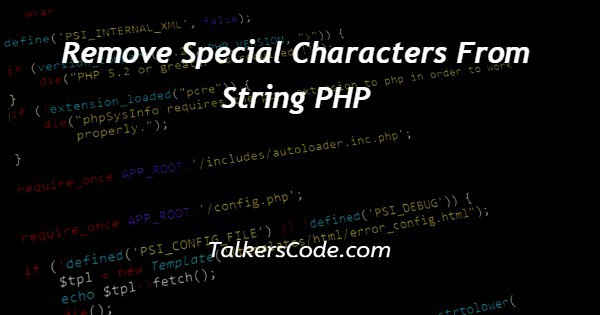# Remove Special Characters From String PHP

Last Updated : Jan 1, 2023In this tutorial we will show you the solution of remove special characters from string PHP, here, for example we get a string from a user and want to remove special characters from that string, then this article is helpful.

Now, let us see how we can done this.

## Step By Step Guide On Remove Special Characters From String PHP :-

In php, there are many ways with help of which we can remove special characters from string. In this article, we are going to use two from them that are

• str_replace()
• preg_replace()

Now, let us see how to use these functions to remove special characters with help of codes.

```<?php
// Function to remove the special characters
function removefunction(\$string) {
// first way – using str_replace() function
\$result = str_replace( array('\'','"',',',';','<','>'),'', \$string);
return \$result;
}
// example is here
\$ string = "Example,to remove<the>Special'Char’ using str_replace() function ;";
\$final_result = removefunction(\$string);
echo \$ final_result;
?>
// another example using another function
<?php
// Function to remove the special characters
function removefunction (\$string){
\$result = preg_replace('/[^a-zA-Z0-9_ -]/s',' ',\$ string);
return \$ result;
}
\$string = "Example,to remove<the>Special'Char using preg_replace() function. is here.;";
\$final_result = removefunction (\$string);
echo \$final_result;
?>```
1. As first of all, let us discuss the purpose of these function that why we use them here.
2. The first function that is str_replace() is used here to remove special characters from string in php. This function generally used to replace the characters with whitespace. Hence, in this way we are able to use remove special characters.
3. Now, in next method that we done using preg_replace() function. It helps us to remove special characters by its nature that is it helps to find out the expression that and we feed and replace them. Hence, in this way we use both these functions to remove special characters.
4. Now, let us understand examples now. Here, as we see that in both examples we create a function in which we give an argument for string and used the required functions inside our custom functions.
5. At last we return the output to show the result to user.
6. Outside this function, as we see that we stored a string inside a variable. We can also use dynamic string here, which means that we have to get string from user and have to store that in a variable. Then we use our custom function on the variable in which we store string. And print the result using echo function.
7. We can also store the result in a variable and then use echo on them to print or display the required output. We hope you understand the above codes easily using these steps. For reference, you can see the above example to see how we done this.

## Conclusion :-

At last in conclusion, here we can say that with the help of this article we are able to understand how to remove special characters from string in php.

I hope this tutorial on remove special characters from string PHP helps you and the steps and method mentioned above are easy to follow and implement.